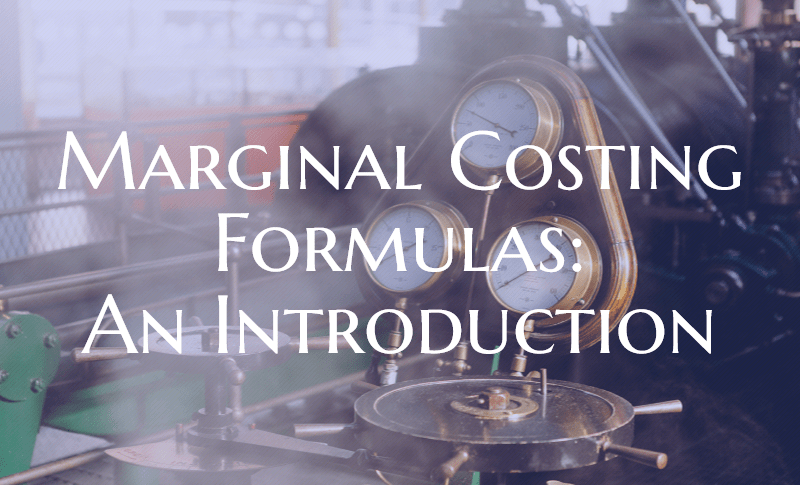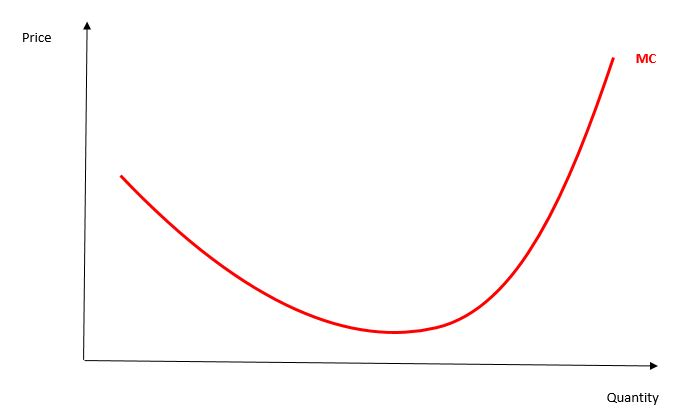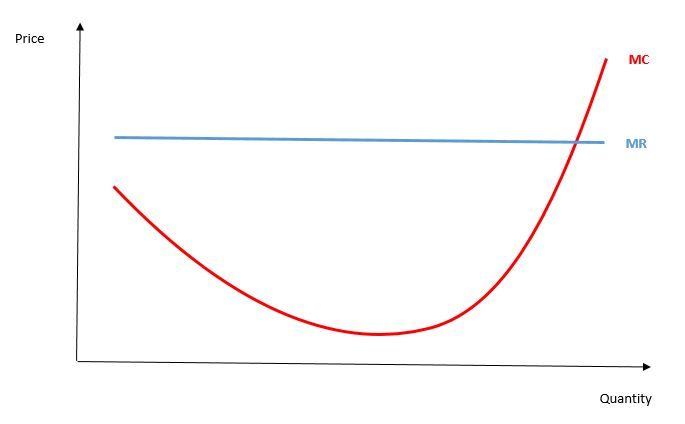# Marginal Costing Formulas: An Introduction

Marginal costing formulas simply show the change in total cost over the change in quantity produced:Normally, marginal cost refers to the amount of cost that will be incurred by an entity in case it produces one extra product. The focus lies in this one extra item.

Imagine, for example, a car manufacturer. Let’s say the company produces 100’000 cars every year and its total cost is \$ 600’000’000. Marginal cost now calculates the extra cost that incurs by producing one more car.Many companies have been using the formula to reduce the cost of production that is being incurred when manufacturing products of different nature and to maximise profits.

## Normal course of marginal costs

In order to better imagine the marginal costs think of it as a graph showing unit costs and numbers of units on its axis. Often you will see a nearly u-shaped curve. Costs start high with a low volume of items but go gradually down as production increases. At some point, however, costs increase again.Whilst economies of scale (fabric utilised to a higher level, discounts for raw material) reduce the marginal cost there will be a point new costs come in and thus the price of an item increases again. Such new costs could be overtime salary, new extra management staff or machinery.

## Advantages of marginal costing formulas

In management accounting theory, there is a famous equation:

marginal cost = marginal revenue

A profit maximising company will produce a product until its marginal costs are equal to the marginal revenue. If marginal revenue would be lower, selling a product would return a loss. Calculating such a point could be a task for a management accountant.In a more complex area, marginal costing can be used to determine the optimum product mix. Imagine a company producing and selling different products. Each of the products will have a separate marginal cost and also separate marginal revenue. It then could be a management accountant’s role to find the best product mix so the company sells many products where the spread between marginal costs and marginal revenue is as high as possible. When doing that we should also keep in mind that limitations of internal resources are likely. There a limiting factor analysis may be required.

## Marginal costs of nearly zero

Nowadays, a special phenomenon can be observed. Some companies can increase their output at a cost of nearly zero. An easy to imagine example is an internet trading platform. If the infrastructure is once up and running, having one extra seller or one extra transaction on it does not bring any extra cost at all.

In this case, marginal costing formulas won’t help you. Instead, finding the most suiting market price will be in focus in order to maximise profits. You can also check the article about Proven cost accounting concepts for more details.

## To sum up

Marginal costing formulas are a valuable tool to determine the output which maximises a company’s profits. This, however, is only a valid for businesses with a certain proportion of variable costs.